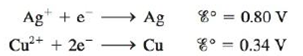# An electrochemical cell consists of a silver metal electrode immersed in a solution with [Ag + ] = 1.00 M separated by a porous disk from a compartment with a copper metal electrode immersed in a solution of 10.00 M NH 3 that also contains 2.4 × 10 −3 M Cu(NH 3 ) 4 2+ . The equilibrium between Cu 2+ and NH 3 is: Cu 2 + ( a q ) + 4 NH 3 ( a q ) ⇌ Cu ( N ​ H 3 ) 4 2 + ( a q ) K = 1.0 × 10 13 and the two cell half-reactions are: Assuming Ag + is reduced, what is the cell potential at 25°C?### Chemistry: An Atoms First Approach

2nd Edition
Steven S. Zumdahl + 1 other
Publisher: Cengage Learning
ISBN: 9781305079243

#### Solutions

Chapter
Section### Chemistry: An Atoms First Approach

2nd Edition
Steven S. Zumdahl + 1 other
Publisher: Cengage Learning
ISBN: 9781305079243
Chapter 17, Problem 138CWP
Textbook Problem
8 views

## An electrochemical cell consists of a silver metal electrode immersed in a solution with [Ag+] = 1.00 M separated by a porous disk from a compartment with a copper metal electrode immersed in a solution of 10.00 M NH3 that also contains 2.4 × 10−3 M Cu(NH3)42+. The equilibrium between Cu2+ and NH3 is: Cu 2 + ( a q )   +   4 NH 3 ( a q )   ⇌ Cu ( N ​ H 3 ) 4 2 + ( a q ) K = 1.0 × 10 13 and the two cell half-reactions are:Assuming Ag+ is reduced, what is the cell potential at 25°C?

Interpretation Introduction

Interpretation:

An electrochemical cell consisting of Silver and Copper electrode dipped in Ammonia solution and its equilibrium constant is given. The value of cell potential at 25°C for the given cell is to be calculated.

Concept introduction:

The relationship between reduction potential and standard reduction potential value and activities of species present in an electrochemical cell at a given temperature is given by the Nernst equation.

The value of Ecell is calculated using Nernst formula,

E=E°(RTnF)ln(Q)

At room temperature, the above equation specifies as,

E=E°(0.0591n)log(Q)

To determine: The value of cell potential at 25°C for the given cell.

### Explanation of Solution

Given,

The concentration of Ag+ is 1.00M .

The concentration of NH3 solution is 1.00M .

The concentration of NH3 solution is 1.00M .

The concentration of [Cu(NH3)4]2+ solution is 2.4×103M .

The value of the equilibrium constant is 1.0×1013

The equilibrium reaction is,

Cu2+(aq)+4NH3(aq)[Cu(NH3)4]2+(aq)

The reaction taking place at the cathode is,

Ag++eAgE°red=0.80V

The reaction taking place at the anode is,

CuCu2++2eE°OX=0.34V

Multiply reduction half-reaction with a coefficient of 2 and then add both the reactions as,

2Ag++2e2AgCuCu2++2e

The final equation is,

2Ag++Cu2Ag+Cu2+

The value of E°cell is given as,

E°cell=E°ox+E°red

Where,

• E°ox is the oxidation potential of the electrode.
• E°red is the reduction potential of the electrode.

Substitute the values of E°ox and E°red in the above equation as,

E°cell=E°ox+E°red=0

### Still sussing out bartleby?

Check out a sample textbook solution.

See a sample solution

#### The Solution to Your Study Problems

Bartleby provides explanations to thousands of textbook problems written by our experts, many with advanced degrees!

Get Started

Find more solutions based on key concepts
Describe diffusion in terms or entropy.

Biology: The Unity and Diversity of Life (MindTap Course List)

After about 50 years of age, bones begin to lose dens.

Nutrition: Concepts and Controversies - Standalone book (MindTap Course List)

Assign IUPAC names to the following alcohols: a. CH3CH2CH2OH b. c. d. e. f.

Chemistry for Today: General, Organic, and Biochemistry

What gives amino acids their individual properties?

Biology: The Dynamic Science (MindTap Course List)

A potters wheela thick stone disk of radius 0.500 m and mass 100 kgis freely rotating at 50.0 rev/min. The pott...

Physics for Scientists and Engineers, Technology Update (No access codes included)

True or false? All traits are inherited in a Mendelian pattern.

Biology: The Unity and Diversity of Life (MindTap Course List)

How does wind blowing over a surface current influence the climate downwind?

Oceanography: An Invitation To Marine Science, Loose-leaf Versin